# Mumford hypothesis

(diff) ← Older revision | Latest revision (diff) | Newer revision → (diff)

The hypothesis that each semi-simple algebraic groupis geometrically reductive, i.e., has the following property: For any rational representation ofin a finite-dimensional vector spaceand any non-zero vector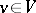fixed by, there is a-invariant homogeneous polynomialof positive degree onfor which.

This hypothesis was stated by D. Mumford  (in a different, but equivalent form) with the aim of finding a property of semi-simple groups, defined over an algebraically closed field of arbitrary characteristic, which, from the point of view of the geometric theory of invariants (cf. Invariants, theory of), would serve as a substitute for the classical property of complete reducibility of rational linear representations of semi-simple groups defined over fields of characteristic zero (this latter property not holding for ground fields of positive characteristic). It would allow the removal of restrictions on the characteristic of the ground field in a number of central results in the geometric theory of invariants, such as the theorem on finite generation of the algebra of invariants of reductive groups of automorphisms of an algebra of finite type over a field (see Hilbert theorem on invariants).

If the characteristic of the ground fieldis zero, then a proof of Mumford's hypothesis is given by Weyl's classical theorem on complete reducibility of rational representations of semi-simple groups (see ): in this case the invariant line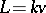inhas an invariant complement(an invariant hyperplane such that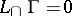), andcan be taken to be the linear form giving the equation of. Whenhas positive characteristic, Mumford's hypothesis generalizes the fact that there is an invariant homogeneous hypersurfaceinfor which(with the degree ofequal to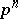for some integer).

Mumford's hypothesis is also equivalent to the assertion that for any regular action of a semi-simple groupon an affine algebraic varietyand for any two closed non-intersecting invariant subsetsandinthere is an invariant regular functiononfor which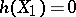and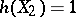(i.e.,and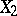can be separated by regular invariants, see ).

Mumford's hypothesis was first proved in ; the proof was extended in  to the general case of reductive group schemes over a field.

The proof of Mumford's hypothesis, together with the results of  and , allow one, first, to give a final form to the generalization of Hilbert's theorem on invariants: Ifis an algebra of finite type over an algebraically closed field,is a reductive group, acting as an automorphism group on, andis the subalgebra of all-invariant elements in, then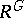is also an algebra of finite type over; and, secondly, to establish that a linear algebraic group over a field of arbitrary characteristic is geometrically reductive if and only if it is reductive (cf. Reductive group). Mumford's hypothesis has applications in the geometric theory of invariants and in moduli theory (see ).

How to Cite This Entry:
Mumford hypothesis. Encyclopedia of Mathematics. URL: http://encyclopediaofmath.org/index.php?title=Mumford_hypothesis&oldid=11673
This article was adapted from an original article by V.L. Popov (originator), which appeared in Encyclopedia of Mathematics - ISBN 1402006098. See original article